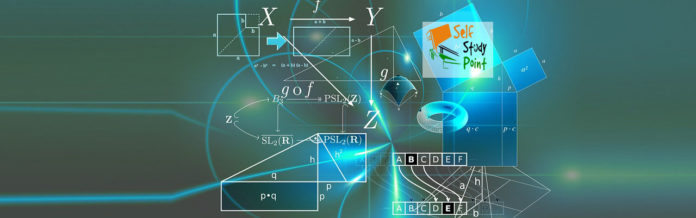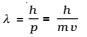The wave nature of light shows up in the phenomena of interference, diffraction and polarization. On the other hand, in photoelectric effect and Compton Effect which involve energy and momentum transfer, radiation behaves as if it is made up of a bunch of particles – the photons.

The gathering and focusing mechanism of light by the eye-lens are well described in the wave picture. But its absorption by the rods and cones (of the retina) requires the photon picture of light.

### De Broglie wavelength:

De Broglie proposed that the wavelength λ associated with a particle of momentum p is given aswhere m is the mass of the particle and v its speed (Eq above ) is known as the de Broglie relation and the wavelength λ of the matter wave is called de Broglie wavelength.

For a photon, as we have seen,That is, the de Broglie wavelength of a photon given by (above λ eq.) equals the wavelength of electromagnetic radiation of which the photon is a quantum of energy and momentum.

The dualism of matter is inherent in the de Broglie relation which contains a wave concept (λ) and a particle concept (p). The de Broglie wavelength is independent of the charge and nature of the material particle. It is significantly measurable (of the order of the atomic-planes spacing in crystals) only in case of sub-atomic particles like electrons, protons, etc. (due to the smallness of their masses and hence, momenta). However, it is indeed very small, quite beyond measurement, in case of macroscopic objects, commonly encountered in everyday life.